# WBJEE Subjective Mathematics Sample Paper I Notes | Study WBJEE Sample Papers, Section Wise & Full Mock Tests - JEE

## JEE: WBJEE Subjective Mathematics Sample Paper I Notes | Study WBJEE Sample Papers, Section Wise & Full Mock Tests - JEE

The document WBJEE Subjective Mathematics Sample Paper I Notes | Study WBJEE Sample Papers, Section Wise & Full Mock Tests - JEE is a part of the JEE Course WBJEE Sample Papers, Section Wise & Full Mock Tests.
All you need of JEE at this link: JEE

Question: 1. The harmonic mean of two numbers is 4. Their arithemetic mean A and the geometric mean G satisfy the relation 2A + G2 = 27. find the numbers.
Ans:
(3, 6)
Solution:  Let the number be a, b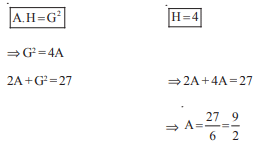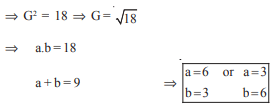Question: 2  If the area of a rectangle is 64 sq. unit, find the minimum value possible for its perimeter.
Ans:
32
Solution: Let the dimesions be a, b
Area = ab
Paimeter = 2(a + b)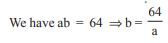Perimeter as function of a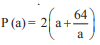for maxima or minimum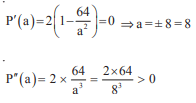P (8) is minimum
Minimum P (8) = 2 ( 8 + 8) = 32

Question: 3. Find the image of the point (–8, 12) with respect to the line 4x + 7y + 13 = 0
Ans:
(–16, –2)
Solution: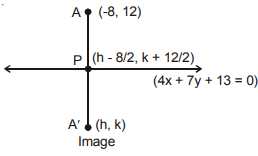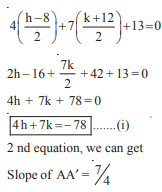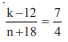⇒ 4k – 48 = 75 + 56
4k – 7h = 104 ........(ii)
Solving (i) & (ii)
Equation (i) × 7 + Equation (ii) × 4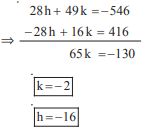A′ (– 16, –2) is the image of (–8, 12)

Question:  4. How many triangles can be formed by joining 6 points lying on a circle ?
Ans: 20
Solution:
Number of triangle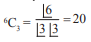Question:  5. If r2 = x2 + y2 + z2 , then prove that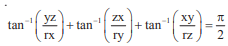Ans: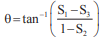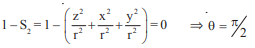Question:  6. Determine the sum of imaginary roots of the equation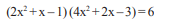Ans: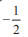Solution:  Put 2x2 + x = y
⇒ (4 – 1) (24 – 3) = 6, on solving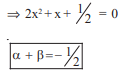Question:  7. If cos A + cos B + cos C = 0, prove that  cos 3A + cos 3B + cos 3C = 12 cos A cos B cos C
Ans: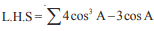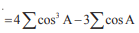= 12 cos A . cos B. cos C

Question: 8.  Let IR be the set of real numbers and f : IR → IR  be such that for all x, y ∈IR, |f(x) – f(y)| ≤ | x – y|3 . Prove that f is a constant function.
Ans: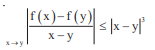= |f′ (x) | ≤ | 0 | ⇒ |f′ (x)| = 0
⇒ f (x) = constant

Question: 9. Find the general solution of (x + logy) dy + y dx = 0
Ans. xy + y lny – y = 0
Solution:  x dy + y dx + log y dy = 0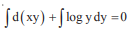xy + y lny – y = 0

Question: 10.  Prove that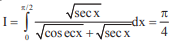Ans.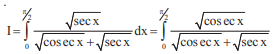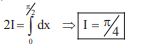The document WBJEE Subjective Mathematics Sample Paper I Notes | Study WBJEE Sample Papers, Section Wise & Full Mock Tests - JEE is a part of the JEE Course WBJEE Sample Papers, Section Wise & Full Mock Tests.
All you need of JEE at this link: JEEUse Code STAYHOME200 and get INR 200 additional OFF

## WBJEE Sample Papers, Section Wise & Full Mock Tests

4 videos|10 docs|54 tests

Track your progress, build streaks, highlight & save important lessons and more!

,

,

,

,

,

,

,

,

,

,

,

,

,

,

,

,

,

,

,

,

,

,

,

,

;